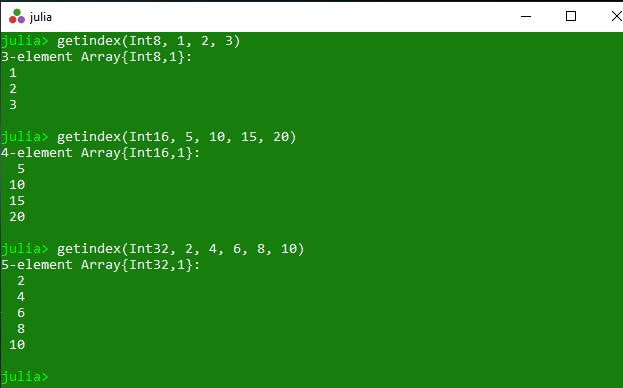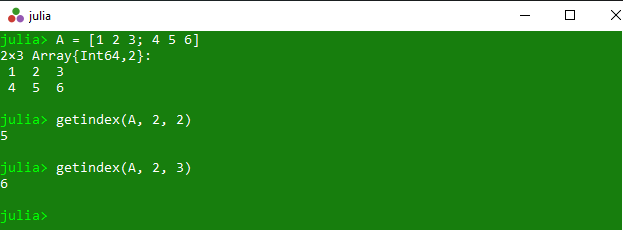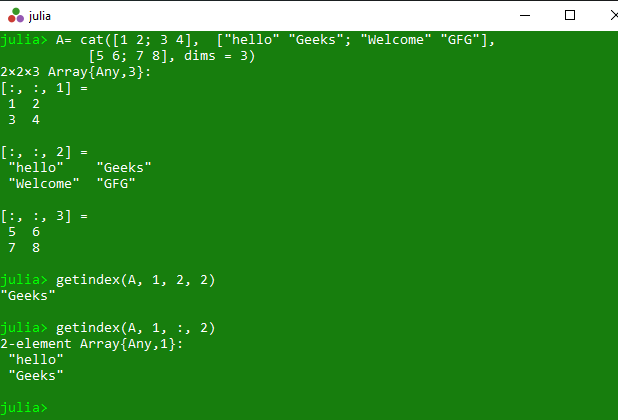# Accessing element at a specific index in Julia – getindex() Method

• Last Updated : 07 Sep, 2021

The getindex() is an inbuilt function in julia which is used to construct array of the specified type. This function is also used to get the element of the array at a specific index.

Syntax: getindex(type, elements…])
Parameters:

• type: Specified type.
• elements: Specified list of elements.

Returns: It returns the constructed array of the specified type and also the element of the array at a specific index.

Example 1:

## Python

 `# Julia program to illustrate``# the use of Array getindex() method` `# Constructing array of the different types``println(getindex(Int8, ``1``, ``2``, ``3``))``println(getindex(Int16, ``5``, ``10``, ``15``, ``20``))``println(getindex(Int32, ``2``, ``4``, ``6``, ``8``, ``10``))`

Output:Example 2:

## Python

 `# Julia program to illustrate``# the use of Array getindex() method` `# Getting an element of the 2D array``# A at some specific indexs of (2, 3)``# and (2, 2)``A ``=` `[``1` `2` `3``; ``4` `5` `6``]``println(getindex(A, ``2``, ``2``))``println(getindex(A, ``2``, ``3``))`

Output:Example 3:

## Python

 `# Julia program to illustrate``# the use of Array getindex() method`` ` `# Getting an element of the 3D array``# A at some specific indexs of (1, 2, 2)``# and (1, :, 2)``A ``=` `cat([``1` `2``; ``3` `4``], ``        ``[``"hello"` `"Geeks"``; ``"Welcome"` `"GFG"``], ``        ``[``5` `6``; ``7` `8``], dims ``=` `3``)``getindex(A, ``1``, ``2``, ``2``)``getindex(A, ``1``, :, ``2``)`

Output:My Personal Notes arrow_drop_up